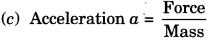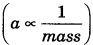# GSEB Solutions Class 9 Science Chapter 9 Force and Laws of Motion

Gujarat Board GSEB Textbook Solutions Class 9 Science Chapter 9 Force and Laws of Motion Textbook Questions and Answers, Notes Pdf.

## Gujarat Board Textbook Solutions Class 9 Science Chapter 9 Force and Laws of Motion

### Gujarat Board Class 9 Science Force and Laws of Motion InText Questions and Answers

Page 118.

Question 1.
Which of the following has more inertia:
(a) rubber and a stone of the same size?
(b) a bicycle and a train?
(c) a five-rupees coin and a one-rupee coin?
(a) Stone has more mass and inertia
(b) A train has greater inertia than a bicycle because the mass of the train is greater than that of the bicycle.
(c) The mass of five rupees coin is greater than the mass of one rupee coin and hence the inertia of five rupees coin is greater.

Question 2.
In the following example, try to identify the number of times the velocity of the ball changes: “A football player kicks a football to another player of his team who kicks the football towards the goal. The goalkeeper of the opposite team collects the football and kicks it towards a player of his own team”. Also, identify the agent supplying the force in each case.
The velocity of football changes four times. First, when a football player kicks to another player. Second, when that player kicks the football to goalkeepers. Third, when the goalkeeper stops the football. Fourth, when the goalkeeper kicks the
football towards a player of his own team. Agent supplying the force
First case – First player
Second case – Second player
Third case – Goalkeeper
Fourth case – GoalkeeperQuestion 3.
Explain why some of the Ieavps may get detached from a tree if we vigorously shake its branch.
When we shake the branch of a tree vigorously, some of the leaves may get detached on account of the inertia of the rest of the leaves. The branch comes in motion and the leaves may get detached on account of inertia of rest of the leaves.

Question 4.
Why do you fall in the forward direction when a moving bus brakes to a stop and fall back when it accelerates from rest?
When moving bus brakes to stop, our feet come to rest with the bus. But the upper part of our body continues to move forward due to the inertia of motion. That is why we tend to fall in the forward direction.

When the bus accelerates from rest, the lower part of our body moves with the bus. The upper part of the body tries to maintain itself at rest due to inertia of rest. Therefore, we tend to fall backward.

Page 126

Question 1.
If action is always equal to the reaction, explain how a horse can pull a cart.
A horse pushes the ground in the backward direction. According to Newton’s third law of motion, a reaction force is exerted by the Earth on the horse in the forward direction. As a result, the cart moves forward.

Question 2.
Explain, why is it difficult for a fireman to hold a hose, which ejects a large amount of water at a high velocity.
When a fireman holds a hose, which is ejecting a large amount of water at high velocity, then a reaction force is exerted on him by the ejecting water in the backward direction. This is because of Newton’s third law of motion. As a result of the backward force, the stability of the fireman decreases. Hence, it is difficult for him to remain stable while holding the hose.

Question 3.
From a rifle of mass 4 kg, a bullet of 50 g is fired with an initial velocity of 35 m/s. Calculate the initial recoil velocity of the rifle.
Given, Mass of rifle, m1 = 4 kg
Mass of bullet, m2 = 50 g = 5 x 10-3 kg
Velocity of bullet, u2 = 35 m/s
Applying principle of conservation of linear momentum,
Pi = Pf
0 = m1u1 + m2u2
= υ1 = – $$\frac{m_{2} v_{2}}{m_{1}}$$
∴ υ1 = – $$\frac{5 \times 10^{-3} \times 35}{4}$$ = 0.044 ms-1
Negative sign indicates that the rifle moves in a direction opposite to the direction of the bullet.Question 4.
Two objects of masses 100 g and 200 g are moving along the same line in the same direction with velocities of 2 m/s and 1 m/s, respectively. They collide and after the collision, the first object moves at a velocity of 1.67 m/s. Determine the velocity of the second object.
Given, Mass of body, m1 = 100 g = 100 x 10-3 kg
Mass of 2nd body, m2 = 200 g = 200 x l0 kg
Initial velocity of 1st body, u1 = 2 m/s
Initial velocity of 2h1 body, u2 = 1 m/s

Final velocity of 1st body, u1 = 1.67 m/s
Applying conservation of linear momentum,
Pi = Pf
m1u1m2u2 = m1u1 + m2v2
(100 x 10-3) x 2 + (200 x 10-3) x 1
= (100 x 10-3) x 1.67 x (200x 10) x υ2
υ2 = 1.165 m/s

In-Text Activities Solved

(Textbook Page 117)

Activity 9.1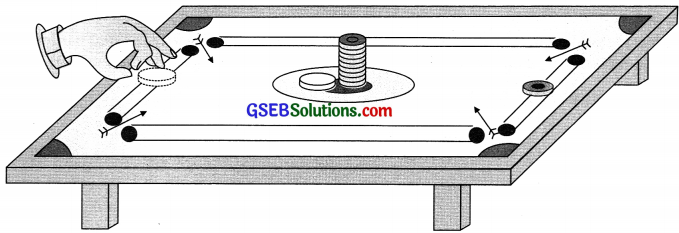Discussion and conclusion
The upper carom coins fall vertically on the table due to the inertia of rest.

(Textbook Page 118)

Activity 9.2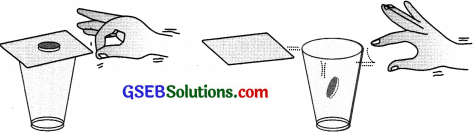Discussion and conclusion
The coin falls vertically into the glass tumbler due to inertia of rest.(Textbook Page 118)

Activity 9.3
Discussion and conclusion
Water from the tumbler spills on account of the inertia of the rest of the water.

(Textbook Page 123)

Activity 9.4Discussion and conclusion
When two children standing on two different carts play the game of catch with the sandbag, we find that the cart of each child moves outwards. This is due to the instantaneous reaction of throwing the bag.

(Textbook Page 124)

Activity 9.5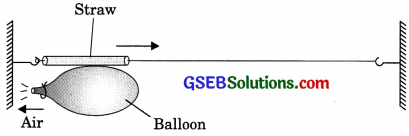Discussion and conclusion
The momentum of the inflated balloon before it is pierced with a pin is zero. When it is pierced with a pin, air in it comes out with a speed in the backward direction. To conserve the momentum, the balloon moves in the forward direction.

(Textbook Page 124)

Activity 9.6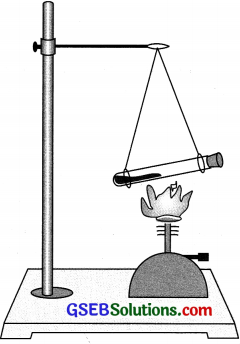Discussion and conclusion
This is due to conservation of momentum. Initially, the momentum of the system is zero. After the cork blows out, steam comes out in one direction, and to conserve momentum, the test tube moves in the opposite direction.

### Gujarat Board Class 9 Science Force and Laws of Motion Textbook Questions and Answers

Question 1.
An object experiences a net zero external unbalanced force. It is possible for the object to be traveling with a non-zero velocity? If yes, state the conditions that must be placed on the magnitude and direction of the velocity. If no, provide a reason.
Yes, an object may travel with a non-zero velocity even when the net external force on it is zero.
The necessary conditions are:

• The object should already be moving with uniform speed along a straight line
• All types of frictional resistance must be zero.

Question 2.
When a carpet is beaten with a stick, dust comes out of it. Explain.
When the carpet is beaten, it is suddenly set into motion. The dust particles tend to remain at rest due to inertia of rest, therefore the dust comes out of it.Question 3.
Why is it advised to tie any luggage kept on the roof of a bus with a rope?
Any luggage kept on the roof of a bus. should be tied with a rope. This is because when the bus starts suddenly, the luggage pieces may fall down due to the inertia of rest. Similarly, when the moving bus stops suddenly, the luggage pieces may fall down due to the inertia of inotion.

Question 4.
A batsman hits a cricket ball which then rolls on level ground. After covering a short distance, the ball comes to rest. The ball slows to a stop because
(a) the batsman did not hit the ball hard enough
(b) velocity is proportional to the force exerted on the ball.
(c) there is a force on the ball opposing the motion.
(d) there is no unbalanced force on the ball, so the ball would want to come to rest.
(c) The cricket ball comes to rest due to the opposition of friction force.

Question 5.
A truck starts from rest and rolls down a hill with a constant acceleration. It travels a distance of 400 m in 20 s. Find its acceleration. Find the force acting on it if its mass is 7 metric tonnes. (Hint: 1 metric tonne = 1000 kg.)
Given, distance, s = 400 m, time t = 20 s
Initial velocity, u = 0, mass, m = 7000 kg
Using equation, s = ut + $$\frac {1}{2}$$ at2
400 = 0 + $$\frac {1}{2}$$ a x 400 = 200 a
∴ a = 2 m/s2
Force acting on body, F = ma = 7000 x 2 = 14000 N.Question 6.
A stone of 1 kg is thrown with a velocity of 20 ms’ across the frozen surface of a lake and comes to rest after traveling a distance of 50 m. What is the force of friction between the stone and the ice?
4nswer:
Given, mass, m = 1 kg; Initial velocity, u = 20 m/s
Distance, s = 50 m
Final velocity, υ = 0 m/s
Applying υ2 – u2 = 2as
⇒ (0)2 – (20)2 = 2 x a x 50
∴ a = 4 m/s2
Force, F = ma
⇒ F = 1 x -4 = – 4N
F = – 4N
Force of friction = 4N

Question 7.
A 8000 kg engine pulls a train of 5 wagons, each of 2000 kg, along a horizontal track. If the engine exerts a force of 40000 N and the track offers a friction force of 5000 N, then calculate:
(a) the net accelerating force;
(b) the acceleration of the train; and
(c) the force of wagon 1 on wagon 2.
Mass of engme, m1 = 8000kg
Mass of all wagons, m2 = 5 x 2000 = 10,000 kg
Force exerted by engine,, F = 40,000 N
Friction force of track, F = 5000 N

(a) Net accelerating force = Force exerted by engine – Frictional force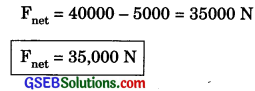(b) Acceleration,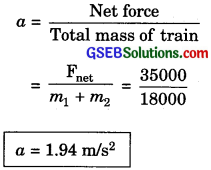(c) Force on wagon 1 on wagon 2
= mass of 4 wagons x acceleration
=4 x 2000 x 1.94
F = 15520 NQuestion 8.
An automobile vehicle has a mass of 1500 kg. What must be the force between the vehicle and road if the vehicle is to be stopped with a negative acceleration of 1.7 ms-2?
Mass of automobile, m = 1500 kg
Acceleration, a = – 1.7 m/s
Force, F = ma
F = 1500 x (- 1.7)
F = – 2550 N

Question 9.
What is the momentum of an object of mass m, moving with a velocity y?
(a) (mυ )2
(b) mυ2
(c) ½ mυ2
(d) mu
(d) momentum = mass x velocity

Question 10.
Using a horizontal force of 200 N, we intend to move a wooden cabinet across a floor at a constant velocity. What is the friction force that will be exerted on the cabinet?
The cabinet will move with constant velocity if the net external force acting on it is zero. Since a horizontal force of 200 N acts on the cabinet in the forward direction, therefore net external force acting on it will be zero if friction force of 200 N acts on it. Thus friction force of 200 N will be exerted on the cabinet.

Question 11.
Two objects, each of mass 1.5 kg, are moving in the same straight line but in opposite directions. The velocity of each object is 2.5 Ms-1 before the collision during which they stick together. What will be the velocity of the combined object after the collision?
Mass of bodies, m1 = m2 = 1.5 kg
Initial velocity of first body, u1 = 2.5 rn/s
Initial velocity of second body,u2 = – 2.5 rn/s
(Negative sign for the opposite direction of motion).
If υ is velocity of the combined object after collision, then applying the principle of conservation of momentum,
(m1 + m2)υ = m1u1 + m2u2
(1.5 + 1.5)υ = 1.5(2.5) + 1.5(- 2.5) = 0
∴ υ = 0Question 12.
According to the third law of motion when we push on an object, the object pushes back on us with an equal and opposite force. If the object is a massive truck parked along the roadside, it will probably not move. A student justifies this by answering that the opposite and equal forces cancel each other. Comment on this logic and explain why the truck does not move.
The student’s justification is not correct. Two equal and opposite forces cancel each other if they act on the same body. According to the third law of motion, action and reaction forces are equal and opposite but they both act on different bodies. Hence, they cannot cancel each other.

When we push a massive truck, then the force applied on the truck is not sufficient to overcome the force of friction between the types of the truck and ground. Hence the truck does not move. The truck will move only if the force applied on it greater than the frictional force.

Question 13.
A hockey ball of mass 200 g traveling at 10 ms is struck by a hockey stick so as to return it along its original path with a velocity at 5 ms. Calculate the change of momentum that occurred in the motion of the hockey ball by the force applied by the hockey stick.
Mass of hockey ball, m = 200 g = 0.2 kg
Initial velocity, u = 10 m/s
Final velocity, u = -5 m/s
Change in momentum = Final momentum – Initial momentum
= mυ – mu =m(υ – u)
= 0.2 (-5- 10) 0.2 x -15
∴ Change in momentum = – 3 kg m/s.

Question 14.
A bullet of mass 10 g traveling horizontally with a velocity of 154) ms1 strikes a stationary wooden block and comes to rest in 0.03 s. Calculate the distance of penetration of the bullet into the block. Also, calculate the magnitude of the force exerted by the wooden block on the bullet.
Mass of bullet, m = 10 g = 10 x 10-3 kg
Initial velocity of bullet, u = 150 m/s
Time, t = 0.03s
Final velocity of bullet, υ = 0
Applying υ = u + at
∴ a = -5 x 103 m/s2
Applying s = ut + $$\frac{1}{2}$$ at2
s = 150 x 0.03 + $$\frac{1}{2}$$ (-5 x 103) (0.03)2
∴ s = 2.25 m
Force exerted, F = ma = 10 x 10-3 (-5 x 103)
∴ F = – 50 NQuestion 15.
An object of mass 1 kg traveling in a straight line with a velocity of 10 m1 collides with and sticks to, a stationary wooden block of mass 5 kg. Then they both move off together in the same straight line. Calculate the total momentum just before the impact and just after the impact. Also, calculate the velocity of the combined object.
Given, Mass of an object, m = 1 kg
Velocity of object, υ = 10 m/s
Mass of wooden block, m = 5 kg
On the basis of conservation of momentum, the initial momentum and final momentum will be the same.
Pi = Pf = mυ = 10 kg m/s
Combined velocity = υ’
Pf = (m + M)υ’
10 = (1 + 5)υ’
υ’= 1.67m/s

Question 16.
An object of mass 100 kg is accelerated uniformly from a velocity of 5 m/s to 8 m/s in 6 s. Calculate the initial and final momentum of the object. Also, find the magnitude of the force exerted on the object.
Given, Mass of object, m = 100 kg
Initial velocity, u = 5 m/s
Final velocity, υ = 8 m/s
Time, t = 6s
Initial momentum of object = mu
= 100 x 5 = 500kg m/s
Final momentum of the object = mu
= 100 x 8 = 800 kg m/s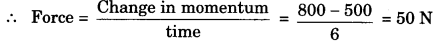Question 17.
Akhtar, Kiran, and Rahul were riding in a motorcar that was moving with a high velocity on an expressway way when an insect hit the windshield and got stuck on the windscreen. Akbar and Kiran started pondering over the situation. Kiran suggested that the insect suffered a greater change in momentum as compared to the change in momentum of the motorcar because the change in the velocity of the insect was much more than that of the motorcar. Akhtar said that since the motorcar was moving with larger velocity, it exerted a larger force on the insect. And as a result, the insect died. Rahul while putting an entirely new explanation said that both the motorcar and the insect experienced the same force and change in their momentum. Comment on these suggestions.
The suggestion made by Iran that the insect suffered a greater change in momentum as compared to the change in momentum of the motor car is wrong. The suggestion made by Akhtar that the motorcar exerted a large force on the insect because of the large velocity of the motorcar is also wrong.The explanation put forward by Rahul that on collision, of the insect with the motorcar, both experience the same force as action and reaction are always equal and opposite i.e., change in momenta of the two occur in opposite directions, though the magnitude of change in momentum of each is the same is correct.

Question 18.
How much momentum will a dumb-bell of mass 10 kg transfer to the floor if it falls from a height of 80 cm? Take its downward acceleration to be 10m/s2.
Given, mass of dumb-bell = 10 kg
Height, h =80 cm = 0.8 m
Acceleration, a = 10 m/s2
initial velocity, u = 0 m/s
Applying υ2 – u2 = 2as
υ2 – 02 = 2 x 10 x 0.8
u2 = 16
∴ υ = 4 m/s
Momentum transferred,
P = mυ = 10 x 4
P = 40 kg m/s.Question 1.
The following is the distance – time table of an object in motion: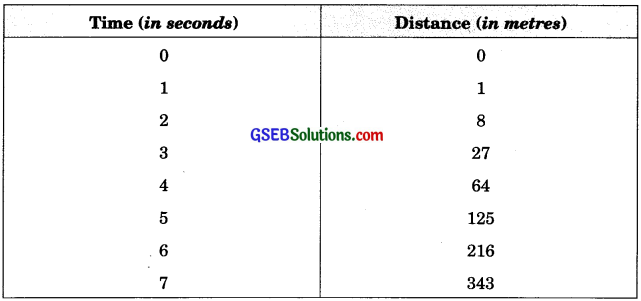1. What conclusion can you draw about the acceleration? It constant, increasing, decreasing, or zero?
2. What do you infer about the forces acting on the object?
1. Initial velocity, u = 0
From the second law of motion,
s = ut + $$\frac {1}{2}$$ at2 = $$\frac {1}{2}$$ at2[∴ u = 0]
a = $$\frac{2 \mathrm{s}}{t^{2}}$$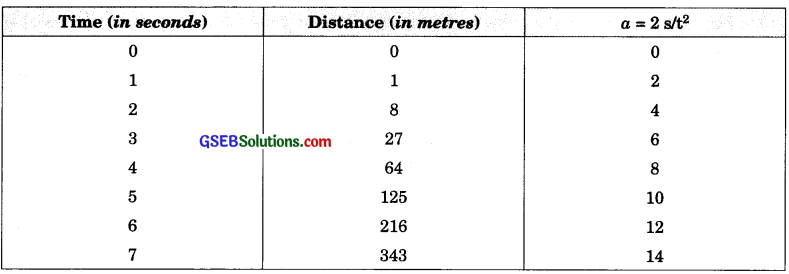Thus, acceleration is increasing.
2. Since acceleration is increasing, so ner unbalanced force is acting on the object.

Question 2.
Two persons manage to push a motorcar of mass 1200 kg at a uniform velocity along a level road. The same motorcar can be pushed by three persons to produce an acceleration of 0.2 m/s2. With what force does each person push the motorcar? (Assume that all persons push the motorcar with the same muscular effort). .
Mass of motorcar, m = 1200 kg
Acceleration, a = 0.2 m/s2
∴ Force applied on the car by three persons, F = ma = 1200 x 0.2 = 240 N
Force applied on the car by one person = = 80 N
Each person pushes the motorcar with a force of 80 N.Question 3.
A hammer of mass 500 g, moving at 50 m/s, strikes a nail. The nail stops the hammer in a very short time of 0.01 s. What is the force of the nail on the hammer?
Mass of the hammer = 500 g = 0.5 kg
Initial velocity of hammer, u = 50 mIs
Final velocity of hammer, υ = 0 [Since the hammer stops]
Time, t = 0.01 s
From the second law of motion,
Force of the nail on the hammer
= rate of change of momentum of the hammer
$$\frac{m v-m u}{t}=\frac{0.5 \times 0-0.5 \times 50}{0.01}$$
= $$\frac{25}{0.01}$$ = – 2500 N
Force of the nail on the hammer is equal and opposite.

Question 4.
A motorcar of mass 1200 kg is moving along a straight line with a uniform velocity of 90 km/h. Its velocity is slowed down to 18 km/h in 4 s by an unbalanced external force. Calculate the acceleration and change in momentum. Also, calculate the magnitude of the force required.
Mass of the motor car, m = 1200 kg
Initial velocity, u = 90 kmih
= 90 x $$\frac{5}{18}$$ = 25 mis
Final velocity, u = 18 kin/h
= 18 x $$\frac{5}{18}$$ = 5 mis
Time, t = 4s

1. ∴ Acceleration, a = $$\frac{v-u}{t}$$ = $$\frac{5-25}{4}$$ = – $$\frac{20}{4}$$ = 5 m/s2 [Negative si indicates that the velocity decreases]

2. ∴ Change in momentum
= Final momentum – Initial momentum
=mu – mu = m(υ-u)
= 1200 (5 – 25)
= 1200 x (-20)
= -24000 kg-m/s

3. ∴ Magnitude of the force required
= Rate of change of momentum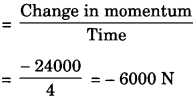Question 5.
A large truck and a car, both moving with a velocity of magnitude y, have a head-on collision and both of them come to a halt after that. If the collision lasts for 1 s:
(a) Which vehicle experiences the greater force of impact?
(b) Which vehicle experiences the greater change in momentum?
(c) Which vehicle experiences the greater acceleration?
(d) Why is the car likely to suffer more damage than the truck?